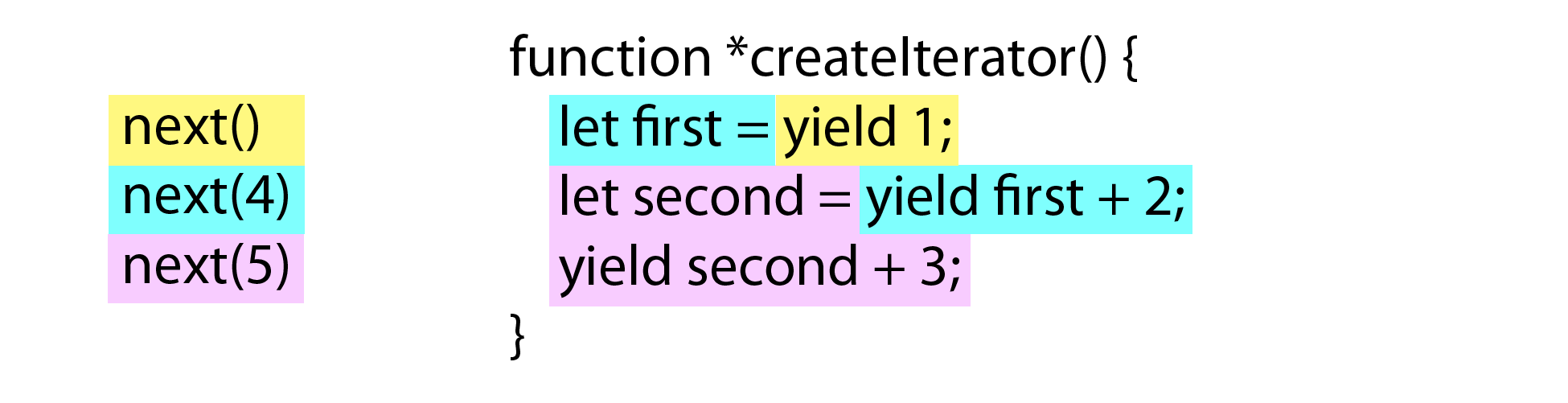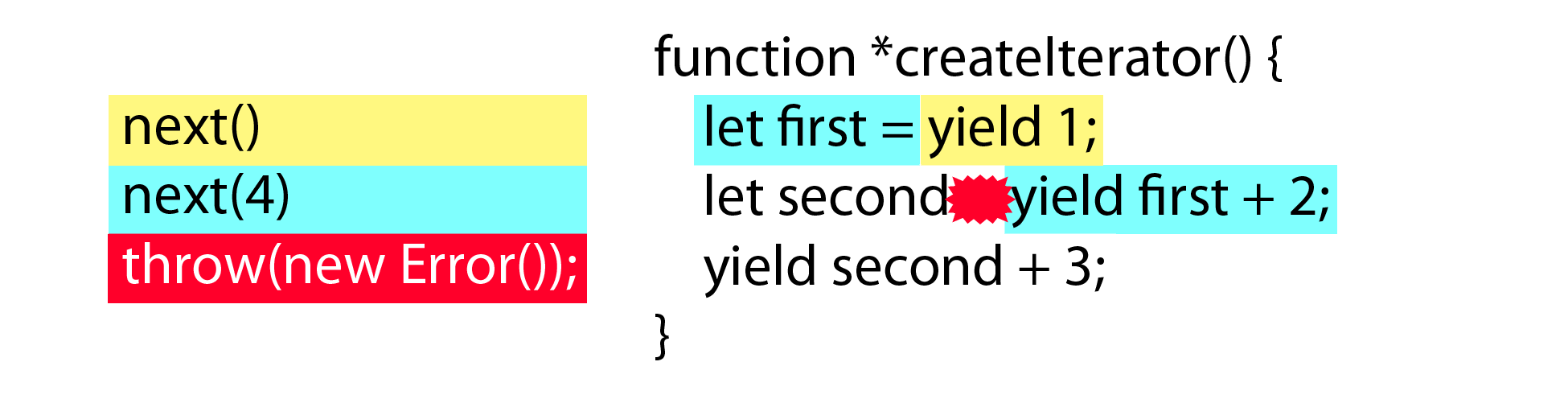# 迭代器与生成器（Iterators and Generators）

### 循环问题（The Loop Problem）

``````var colors = ["red", "green", "blue"];

for (var i = 0, len = colors.length; i < len; i++) {
console.log(colors[i]);
}
``````

### 什么是迭代器（What are Iterators?）

``````function createIterator(items) {

var i = 0;

return {
next: function() {

var done = (i >= items.length);
var value = !done ? items[i++] : undefined;

return {
done: done,
value: value
};

}
};
}

var iterator = createIterator([1, 2, 3]);

console.log(iterator.next());           // "{ value: 1, done: false }"
console.log(iterator.next());           // "{ value: 2, done: false }"
console.log(iterator.next());           // "{ value: 3, done: false }"
console.log(iterator.next());           // "{ value: undefined, done: true }"

// for all further calls
console.log(iterator.next());           // "{ value: undefined, done: true }"
``````

createIterator() 函数返回一个带有 next() 方法的对象。每次调用该方法时，数组中下一位置的值会传给 value 并返回它。当 i 递增为 3 之后，返回的对象中 done 属性为 true，而 value 的值则是三元运算符的计算结果：undefind 。在数据集末尾之后的迭代，即末尾的数据返回之后再调用 next()，这两个属性值的结果也符合 ECMAScript 6 中的迭代器的规范。

### 什么是生成器（What Are Generators?）

``````// 生成器
function *createIterator() {
yield 1;
yield 2;
yield 3;
}

// 调用生成器类似于调用函数，但是前者返回一个迭代器
let iterator = createIterator();

console.log(iterator.next().value);     // 1
console.log(iterator.next().value);     // 2
console.log(iterator.next().value);     // 3
``````

createIterator() 前面的星号指示该函数是个生成器。ECMAScript 6 新引入的 yield 关键字指定迭代器调用 next() 时按顺序返回的值。本例中的生成的迭代器在 next() 方法调用后成功地返回了三个值：先是 1，接着是 2，最后是 3 。一个生成器可以被当做函数调用并创建迭代器。

yield 关键字可以和值或者是表达式一起使用，所以你可以通过生成器给迭代器添加一些项而并非手动地让迭代器一个接一个地返回它们。例如，下面演示了在 for 循环内部使用 yield：

``````function *createIterator(items) {
for (let i = 0; i < items.length; i++) {
yield items[i];
}
}

let iterator = createIterator([1, 2, 3]);

console.log(iterator.next());           // "{ value: 1, done: false }"
console.log(iterator.next());           // "{ value: 2, done: false }"
console.log(iterator.next());           // "{ value: 3, done: false }"
console.log(iterator.next());           // "{ value: undefined, done: true }"

// 进一步调用
console.log(iterator.next());           // "{ value: undefined, done: true }"
``````

``````function *createIterator(items) {

items.forEach(function(item) {

// 语法错误
yield item + 1;
});
}
``````

#### 生成器函数表达式（Generator Function Expressions）

``````let createIterator = function *(items) {
for (let i = 0; i < items.length; i++) {
yield items[i];
}
};

let iterator = createIterator([1, 2, 3]);

console.log(iterator.next());           // "{ value: 1, done: false }"
console.log(iterator.next());           // "{ value: 2, done: false }"
console.log(iterator.next());           // "{ value: 3, done: false }"
console.log(iterator.next());           // "{ value: undefined, done: true }"

// 进一步调用
console.log(iterator.next());           // "{ value: undefined, done: true }"
``````

#### 对象中的生成器方法（Generator Object Methods）

``````var o = {

createIterator: function *(items) {
for (let i = 0; i < items.length; i++) {
yield items[i];
}
}
};

let iterator = o.createIterator([1, 2, 3]);
``````

``````var o = {

*createIterator(items) {
for (let i = 0; i < items.length; i++) {
yield items[i];
}
}
};

let iterator = o.createIterator([1, 2, 3]);
``````

### 可迭代类型与 for-of（Iterables and for-of）

for-lof 循环会在可迭代类型每次迭代执行后调用 next() 并将结果对象存储在变量中。循环会持续进行直到结果对象的 done 属性为 true。如下所示：

``````let values = [1, 2, 3];

for (let num of values) {
console.log(num);
}
``````

This code outputs the following:

``````1
2
3
``````

for-of 循环首先会调用 values 数组的 Symbol.iterator 方法来获取迭代器（Symbol.iterator 方法由幕后的 JavaScript 引擎调用）。之后再调用 iterator.next() 并将结果对象中的 value 属性值，即 1，2，3，依次赋给 num 变量。当检测到结果对象中的 done 为 true，循环会退出，所以 num 不会被赋值为 undefined 。

#### 访问默认迭代器（Accessing the Default Iterator）

``````let values = [1, 2, 3];
let iterator = values[Symbol.iterator]();

console.log(iterator.next());           // "{ value: 1, done: false }"
console.log(iterator.next());           // "{ value: 2, done: false }"
console.log(iterator.next());           // "{ value: 3, done: false }"
console.log(iterator.next());           // "{ value: undefined, done: true }"
``````

``````function isIterable(object) {
return typeof object[Symbol.iterator] === "function";
}

console.log(isIterable([1, 2, 3]));     // true
console.log(isIterable("Hello"));       // true
console.log(isIterable(new Map()));     // true
console.log(isIterable(new Set()));     // true
console.log(isIterable(new WeakMap())); // false
console.log(isIterable(new WeakSet())); // false
``````

isIterable() 函数简单地查看对象是否有默认的并且类型为函数的迭代器。for-of 在执行前也会做相似的检查。

#### 创建可迭代类型（Creating Iterables）

``````let collection = {
items: [],
*[Symbol.iterator]() {
for (let item of this.items) {
yield item;
}
}

};

collection.items.push(1);
collection.items.push(2);
collection.items.push(3);

for (let x of collection) {
console.log(x);
}
``````

``````1
2
3
``````

### 内置的迭代器（Built-in Iterators）

#### 集合迭代器（Collection Iterators）

ECMAScript 6 内置了三种类型的集合对象：数组，map 和 set 。它们都有如下内置的迭代器供你浏览数据。

• entries() - 返回一个数据集为集合中的键值对的迭代器
• values() - 返回一个数据集为集合中的值的迭代器
• keys() - 返回一个数据集为集合中的键的迭代器

##### entries() 迭代器（The entries() Iterator）

``````let colors = [ "red", "green", "blue" ];
let tracking = new Set([1234, 5678, 9012]);
let data = new Map();

data.set("title", "Understanding ECMAScript 6");
data.set("format", "ebook");

for (let entry of colors.entries()) {
console.log(entry);
}

for (let entry of tracking.entries()) {
console.log(entry);
}

for (let entry of data.entries()) {
console.log(entry);
}
``````

console.log() 会做如下输出：

``````[0, "red"]
[1, "green"]
[2, "blue"]
[1234, 1234]
[5678, 5678]
[9012, 9012]
["title", "Understanding ECMAScript 6"]
["format", "ebook"]
``````

##### values() 迭代器（The values() Iterator）

values() 迭代器简单地返回了集合中每一项的值，正如它们在集合中的表现的那样，例如：

``````let colors = [ "red", "green", "blue" ];
let tracking = new Set([1234, 5678, 9012]);
let data = new Map();

data.set("title", "Understanding ECMAScript 6");
data.set("format", "ebook");

for (let value of colors.values()) {
console.log(value);
}

for (let value of tracking.values()) {
console.log(value);
}

for (let value of data.values()) {
console.log(value);
}
``````

``````"red"
"green"
"blue"
1234
5678
9012
"Understanding ECMAScript 6"
"ebook"
``````

##### keys() 迭代器（the keys() Iterator）

keys() 迭代器返回集合中每一项的键。其中数组除了数字索引之外不会返回其它属性。由于 set 中的键和值相同，所以 keys() 和 values() 返回了相同的迭代器。对于 map 来讲，keys() 迭代器会返回每一项中的键。以下示例演示了这三种集合：

``````let colors = [ "red", "green", "blue" ];
let tracking = new Set([1234, 5678, 9012]);
let data = new Map();

data.set("title", "Understanding ECMAScript 6");
data.set("format", "ebook");

for (let key of colors.keys()) {
console.log(key);
}

for (let key of tracking.keys()) {
console.log(key);
}

for (let key of data.keys()) {
console.log(key);
}
``````

``````0
1
2
1234
5678
9012
"title"
"format"
``````

keys() 迭代器获取了 colors，tracking 和 data 各自所有的键，并将它们在 for-of 中打印输出。数组对象只会有索引数字输出，即使你尝试给数组添加命名属性也无济于事。这和 for-in 循环有些不同，因为 for-in 会迭代数组所有的属性而不仅仅是数字索引。

##### 集合类型的默认迭代器（Default Iterators for Collection Types）

``````let colors = [ "red", "green", "blue" ];
let tracking = new Set([1234, 5678, 9012]);
let data = new Map();

data.set("title", "Understanding ECMAScript 6");
data.set("format", "print");

// 等效于调用 colors.values()
for (let value of colors) {
console.log(value);
}

// 等效于调用 tracking.values()
for (let num of tracking) {
console.log(num);
}

// 等效于调用 using data.entries()
for (let entry of data) {
console.log(entry);
}
``````

``````"red"
"green"
"blue"
1234
5678
9012
["title", "Understanding ECMAScript 6"]
["format", "print"]
``````

#### 解构与 for-of 循环（Destructuring and for-of Loops）

Map 构造函数的默认行为有助于在 for-of 循环中使用解构。如下所示：

``````let data = new Map();

data.set("title", "Understanding ECMAScript 6");
data.set("format", "ebook");

// 等效于调用 data.entries()
for (let [key, value] of data) {
console.log(key + "=" + value);
}
``````

#### 字符串迭代器（String Iterators）

``````var message = "A ð ®· B";

for (let i=0; i < message.length; i++) {
console.log(message[i]);
}
``````

``````A
(blank)
(blank)
(blank)
(blank)
B
``````

``````var message = "A ð ®· B";

for (let c of message) {
console.log(c);
}
``````

``````A
(blank)
ð ®·
(blank)
B
``````

#### NodeList 的迭代器（NodeList Iterators）

``````var divs = document.getElementsByTagName("div");

for (let div of divs) {
console.log(div.id);
}
``````

### 扩展运算符与非数组可迭代类型（The Spread Operator and Non-Array Iterables）

``````let set = new Set([1, 2, 3, 3, 3, 4, 5]),
array = [...set];

console.log(array);             // [1,2,3,4,5]
``````

``````let map = new Map([ ["name", "Nicholas"], ["age", 25]]),
array = [...map];

console.log(array);         // [ ["name", "Nicholas"], ["age", 25]]
``````

``````let smallNumbers = [1, 2, 3],
bigNumbers = [100, 101, 102],
allNumbers = [0, ...smallNumbers, ...bigNumbers];

console.log(allNumbers.length);     // 7
console.log(allNumbers);    // [0, 1, 2, 3, 100, 101, 102]
``````

#### 向迭代器传入参数（Passing Arguments to Iterators）

``````function *createIterator() {
let first = yield 1;
let second = yield first + 2;       // 4 + 2
yield second + 3;                   // 5 + 3
}

let iterator = createIterator();

console.log(iterator.next());           // "{ value: 1, done: false }"
console.log(iterator.next(4));          // "{ value: 6, done: false }"
console.log(iterator.next(5));          // "{ value: 8, done: false }"
console.log(iterator.next());           // "{ value: undefined, done: true }"
``````#### 在迭代器中抛出错误（Throwing Errors in Iterators）

``````function *createIterator() {
let first = yield 1;
let second = yield first + 2;       // yield 4 + 2, 之后抛出错误
yield second + 3;                   // 不会执行
}

let iterator = createIterator();

console.log(iterator.next());                   // "{ value: 1, done: false }"
console.log(iterator.next(4));                  // "{ value: 6, done: false }"
console.log(iterator.throw(new Error("Boom"))); // 由生成器抛出错误
````````````function *createIterator() {
let first = yield 1;
let second;

try {
second = yield first + 2;       // yield 4 + 2, then throw
} catch (ex) {
second = 6;                     // 有错误发生，给 second 赋另外的值
}
yield second + 3;
}

let iterator = createIterator();

console.log(iterator.next());                   // "{ value: 1, done: false }"
console.log(iterator.next(4));                  // "{ value: 6, done: false }"
console.log(iterator.throw(new Error("Boom"))); // "{ value: 9, done: false }"
console.log(iterator.next());                   // "{ value: undefined, done: true }"
``````

next() 和 throw() 方法根据 yield 来控制迭代器内部的执行，其实 return 语句也是可以使用的。不过 return 的行为和在普通函数中不太一样，下一节你会看到它们的差异。

#### 包含 return 语句的生成器（Generator Return Statements）

``````function *createIterator() {
yield 1;
return;
yield 2;
yield 3;
}

let iterator = createIterator();

console.log(iterator.next());           // "{ value: 1, done: false }"
console.log(iterator.next());           // "{ value: undefined, done: true }"
``````

``````function *createIterator() {
yield 1;
return 42;
}

let iterator = createIterator();

console.log(iterator.next());           // "{ value: 1, done: false }"
console.log(iterator.next());           // "{ value: 42, done: true }"
console.log(iterator.next());           // "{ value: undefined, done: true }"
``````

#### 生成器代理（Delegating Generators）

``````function *createNumberIterator() {
yield 1;
yield 2;
}

function *createColorIterator() {
yield "red";
yield "green";
}

function *createCombinedIterator() {
yield *createNumberIterator();
yield *createColorIterator();
yield true;
}

var iterator = createCombinedIterator();

console.log(iterator.next());           // "{ value: 1, done: false }"
console.log(iterator.next());           // "{ value: 2, done: false }"
console.log(iterator.next());           // "{ value: "red", done: false }"
console.log(iterator.next());           // "{ value: "green", done: false }"
console.log(iterator.next());           // "{ value: true, done: false }"
console.log(iterator.next());           // "{ value: undefined, done: true }"
``````

``````function *createNumberIterator() {
yield 1;
yield 2;
return 3;
}

function *createRepeatingIterator(count) {
for (let i=0; i < count; i++) {
yield "repeat";
}
}

function *createCombinedIterator() {
let result = yield *createNumberIterator();
yield *createRepeatingIterator(result);
}

var iterator = createCombinedIterator();

console.log(iterator.next());           // "{ value: 1, done: false }"
console.log(iterator.next());           // "{ value: 2, done: false }"
console.log(iterator.next());           // "{ value: "repeat", done: false }"
console.log(iterator.next());           // "{ value: "repeat", done: false }"
console.log(iterator.next());           // "{ value: "repeat", done: false }"
console.log(iterator.next());           // "{ value: undefined, done: true }"
``````

``````function *createNumberIterator() {
yield 1;
yield 2;
return 3;
}

function *createRepeatingIterator(count) {
for (let i=0; i < count; i++) {
yield "repeat";
}
}

function *createCombinedIterator() {
let result = yield *createNumberIterator();
yield result;
yield *createRepeatingIterator(result);
}

var iterator = createCombinedIterator();

console.log(iterator.next());           // "{ value: 1, done: false }"
console.log(iterator.next());           // "{ value: 2, done: false }"
console.log(iterator.next());           // "{ value: 3, done: false }"
console.log(iterator.next());           // "{ value: "repeat", done: false }"
console.log(iterator.next());           // "{ value: "repeat", done: false }"
console.log(iterator.next());           // "{ value: "repeat", done: false }"
console.log(iterator.next());           // "{ value: undefined, done: true }"
``````

``````let fs = require("fs");

if (err) {
throw err;
}

doSomethingWith(contents);
console.log("Done");
});
``````

fs.readFile() 方法包含 filename 参数和一个回调函数。当该操作完成后，回调函数开始执行。回调函数会检查是否有错误发生，如果没有问题则会处理返回的相应内容。在异步任务简单且有限的情况下，这种实现还算可以，一旦需要嵌套多个回调函数或者处理一大批异步任务时，代码会变得极其复杂。生成器和 yield 正好解决了这个问题。

``````function run(taskDef) {

// 创建迭代器，使它们可以在别处使用

// 任务开始执行

// 递归函数持续调用 next()
function step() {

// 如果任务未完成
if (!result.done) {
step();
}
}

// 开始递归
step();

}
``````

run() 函数接收一个已定义的任务（生成器函数）作为参数。该函数内部调用生成器来创建迭代器并将其存储在 task 中。task 变量在（step）函数外部，所以它能被其它函数访问；本小节后面我会解释缘由。首次调用 next() 令迭代器开始运行并存储其结果以便之后使用。step() 函数检查 result.done 是否为 false，如果答案为是那么在递归自身之前先调用 next() 方法。每次调用 next() 都会将结果存储在 result 中，它总是会被最新的信息覆盖。首次调用 step() 会开始递归并查看 result.done 以判断是否还有更多的工作要做。

``````run(function*() {
console.log(1);
yield;
console.log(2);
yield;
console.log(3);
});
``````

``````function run(taskDef) {

// 创建迭代器，使它们可以在别处使用

// 任务开始执行

// 递归函数持续调用 next()
function step() {

// 如果任务未完成
if (!result.done) {
step();
}
}

// 开始递归
step();

}
``````

``````run(function*() {
let value = yield 1;
console.log(value);         // 1

value = yield value + 3;
console.log(value);         // 4
});
``````

Here’s one way you might signal that a value is an asynchronous operation:

``````function fetchData() {
return function(callback) {
callback(null, "Hi!");
};
}
``````

``````function fetchData() {
return function(callback) {
setTimeout(function() {
callback(null, "Hi!");
}, 50);
};
}
``````

``````function run(taskDef) {

// 创建迭代器，使它们可以在别处使用

// 任务开始执行

// 递归函数持续调用 next()
function step() {

// 如果任务未完成
if (!result.done) {
if (typeof result.value === "function") {
result.value(function(err, data) {
if (err) {
return;
}

step();
});
} else {
step();
}

}
}

// 开始递归
step();

}
``````

``````let fs = require("fs");

return function(callback) {
};
}
``````

``````run(function*() {
doSomethingWith(contents);
console.log("Done");
});
``````

### 总结（Summary）

for-of 循环使用可迭代类型来循环返回一系列的元素。相比传统的 for 循环，for-of 在迭代上更为简单，因为你无需跟踪索引值和设定循环结束条件。for-of 循环会自动读取迭代器返回的值并在没有多余项可供迭代的情况下退出。